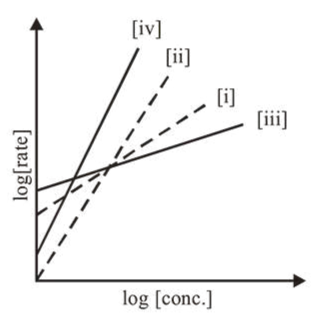# Consider the following reactionsQuestion:

Consider the following reactions $\mathrm{A} \rightarrow \mathrm{P} 1 ; \mathrm{B} \rightarrow \mathrm{P} 2 ; \mathrm{C} \rightarrow \mathrm{P} 3 ; \mathrm{D} \rightarrow \mathrm{P} 4$ The order of the above reactions are (i), (ii), (iii), and (iv), respectively. The following graph is obtained when $\log$ [rate] vs. $\log$ [conc.] are plotted :Among the following, the correct sequence for the order of the reactions is:

1. (iv) $>$ (i) $>$ (ii) $>$ (iii)

2. (i) $>$ (ii) $>$ (iii) $>$ (iv)

3. (iii) $>$ (i) $>$ (ii) $>$ (iv)

4. (iv) $>$ (ii) $>$ (i) $>$ (iii)

Correct Option: , 4

Solution:

Rate $=k[\mathrm{~A}]^{n}$

$\log [$ Rate $]=\log k+n \log [\mathrm{A}]$

Slope $=n[n$ is order of the reaction $]$

$\therefore$ Correct sequence for the order of the reaction is

(iv) $>$ (ii) $>$ (i) $>$ (iii)